# One-4-All: Neural Potential Fields for Embodied Navigation

#### IEEE/RSJ International Conference on Intelligent Robots and Systems (IROS 2023)

Sacha Morin*
DIRO, Mila - Quebec AI Institute
Université de Montréal
Miguel Saavedra-Ruiz*
DIRO, Mila - Quebec AI Institute
Université de Montréal
Liam Paull
DIRO, Mila - Quebec AI Institute
Université de Montréal

*Authors contributed equally.

Abstract. A fundamental task in robotics is to navigate between two locations. In particular, real-world navigation can require long-horizon planning using high-dimensional RGB images, which poses a substantial challenge for end-to-end learning-based approaches. Current semi-parametric methods instead achieve long-horizon navigation by combining learned modules with a topological memory of the environment, often represented as a graph over previously collected images. However, using these graphs in practice requires tuning a number of pruning heuristics. These heuristics are necessary to avoid spurious edges, limit runtime memory usage and maintain reasonably fast graph queries in large environments. In this work, we present One-4-All (O4A), a method leveraging self-supervised and manifold learning to obtain a graph-free, end-to-end navigation pipeline in which the goal is specified as an image. Navigation is achieved by greedily minimizing a potential function defined continuously over image embeddings. Our system is trained offline on non-expert exploration sequences of RGB data and controls, and does not require any depth or pose measurements. We show that O4A can reach long-range goals in 8 simulated Gibson indoor environments and that resulting embeddings are topologically similar to ground truth maps, even if no pose is observed. We further demonstrate successful real-world navigation using a Jackal UGV platform.

This page aims to present an overview of our method, as well as additional videos, figures and experiment details. Have a look at our paper for an in-depth presentation of the method!

We consider a robot with a discrete action space $\actions = \{\mathtt{STOP}, \mathtt{FORWARD}, \mathtt{ROTATE\_ RIGHT}, \mathtt{ROTATE\_ LEFT}\}$ for an image-goal navigation task. Using our knowledge of the robot's geometry and an appropriate exteroceptive onboard sensor (e.g., a front laser scanner), we assume that the set of collision-free actions $\freeactions$ can be estimated. When prompted with a goal image, the agent should navigate to the goal location in a partially observable setting using only RGB observations $\obs_t$ and the $\freeactions$ estimates. The agent further needs to identify when the goal has been reached by autonomously calling the $\mathtt{STOP}$ action in the vicinity of the goal.

## Method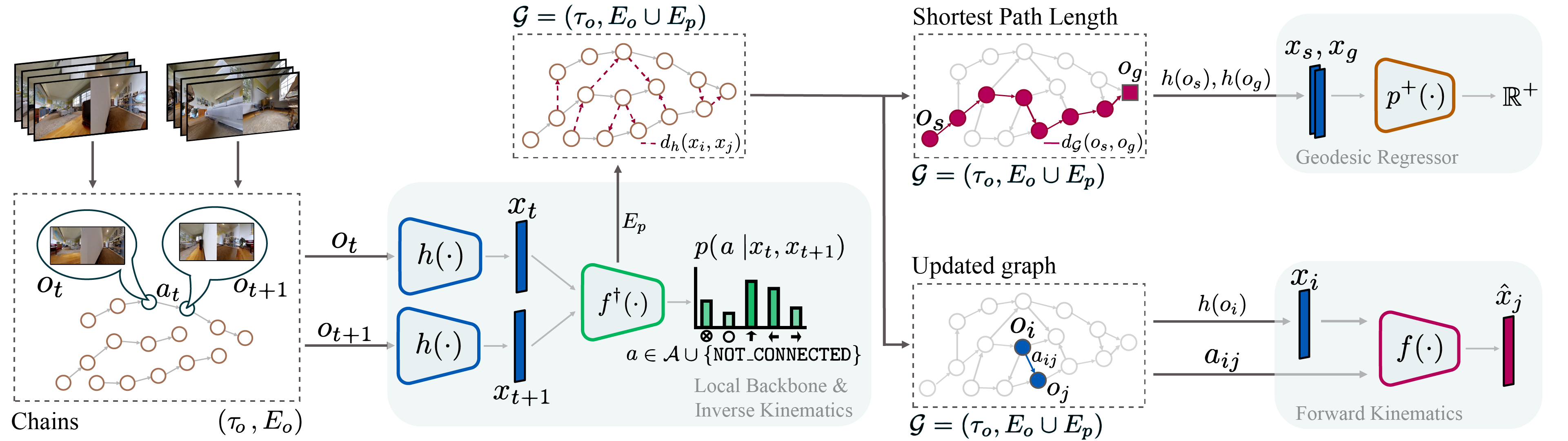O4A consists of 4 learnable deep networks trained with previously collected RGB observation trajectories $\tau_{\obs} =\{\obs_t\}_{t=1}^T$ and corresponding actions $\actiontraj = \{a_t\}_{t=1}^T$, without pose:

• The local backbone $\local$ (left) takes as input RGB images to produce low-dimensional latent embeddings $\code \in \latentspace$ and is trained with a self-supervised time contrastive objective. Once trained, the local backbone can output a local metric $\norm{\code_t - \code_s}$ to measure similarity between observations. The extracted embeddings will also be used as inputs for other modules;
• The inverse kinematics head $\conn$ (center) uses pairs of embeddings to predict the action required to traverse from one embedding to the other (order matters), or the inability to do so through the $\mathtt{NOT\_CONNECTED}$ output;

$\local$ and $\conn$ can then perform loop closures over $\tau_{\obs}$ and construct a directed graph $\graph$, where nodes represent images. Edges represent one-action traversability and are weighted using the local metric. $\graph$ will not be required for navigation, and is only relied on to derive training objectives for the last two components:

• The forward kinematics head (bottom right) $\fd$ is trained using edges from $\graph$ to predict the next embedding $\code_j$ given the current embedding $\code_i$ and an action $a_{ij} \in \actions$;
• The geodesic regressor $\georeg$ (top right), which learns to predict the shortest path length between images embeddings. $\georeg$ is the core planning module and can be interpreted as encoding the geometry of $\graph$.

When multiple environments are considered, $\local$, $\conn$ and $\fd$ are shared across environments, and we train environment-specific regressors $\georeg_i$.

The geodesic regressor $\georeg$ provides a powerful signal to navigate to a goal image (green goal marker). Indeed, it factors in the environment geometry and can, for example, drive an agent out of a dead end to reach a goal that is close in terms of Euclidean distance, but far geodesically. We use $\georeg$ as the attractor in a potential function $\mathcal{P}$ in tandem with repulsors $p^{-}$ around previously visited observations (red markers). At each step, the agent picks the action that leads to a potential-minimizing waypoint W. While we illustrate the potential function on the map, it is in fact defined directly over image embeddings in $\latentspace$.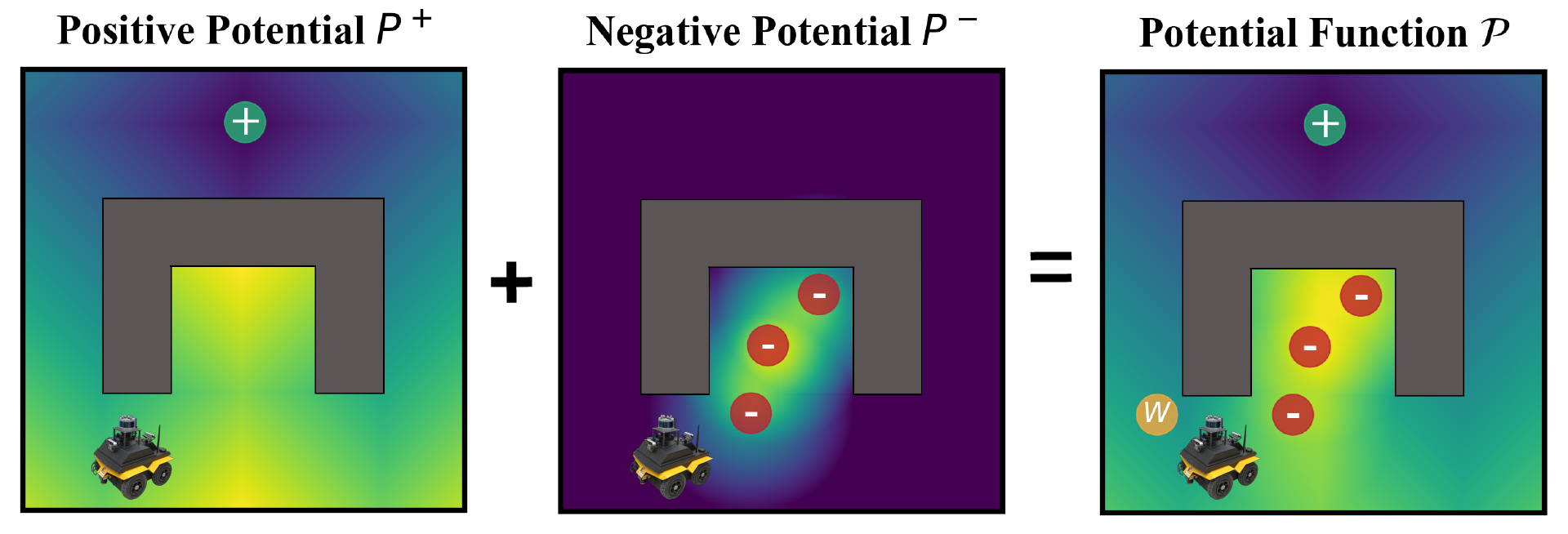Furthermore, we call $\mathtt{STOP}$ by thresholding the local metric between the current image and the goal image. We found this to be more reliable than relying on $\conn$.

## Simulation Experiments

We perform our simulation experiments using 8 scenes from the Gibson dataset rendered with the Habitat simulator. Trajectories are categorized into easy (1.5 $-$ 3m), medium (3 $-$ 5m), hard (5 $-$ 10m) and very hard (> 10m) based on their geodesic distance to the goal. The agent is a differential drive robot with two RGB cameras, one facing forward and the other facing backward. We showcase O4A trajectories for all scenes (rows) and difficulty levels (columns).

Aloha

Annawan

Cantwell

Dunmor

Eastville

Hambleton

Nicut

Sodaville

We compare O4A with relevant baselines and further study two additional O4A variants by ablating terms in the potential function $\mathcal{P}$. We also denote which methods rely on a graph ($\graph$) for navigation and oracle stopping (other methods need to call $\mathtt{STOP}$ autonomously). We find that O4A substantially outperforms baselines, achieving a higher Success Rate (SR), Soft Success Rate (SSR), Success Weighted by Path Length (SPL), and a competitive ratio of Collision-Free Trajectories (CFT). The two O4A ablations confirm that all considered potentials in our potential function $\mathcal{P}$ are essential contributors to success.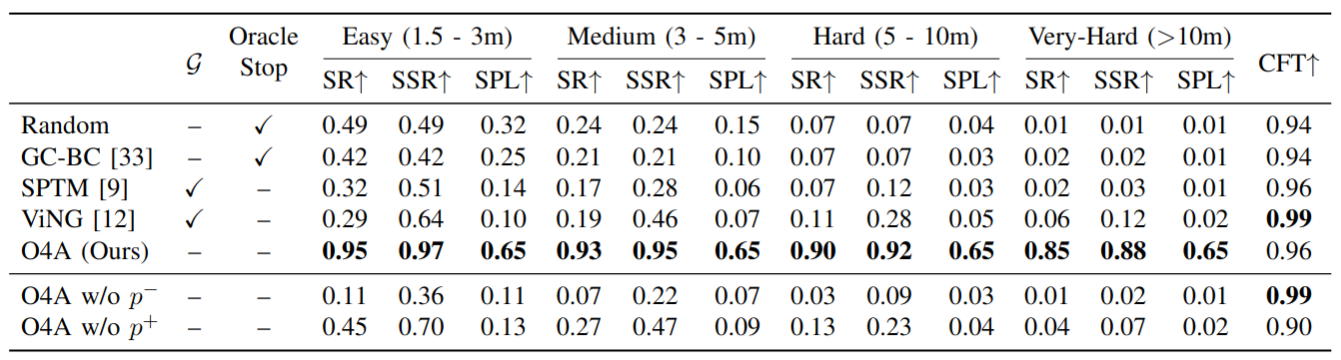## Robot Experiments

For the real-world experiments we run O4A on the Clearpath Jackal platform over 9 episodes in our lab, repeated 3 times each. A video with 2 robot episodes is shown at the top of the page. In addition to Success Rate (SR) and Soft Success Rate (SSR), we evaluate the final distance to goal (DTG), the number of $\mathtt{FORWARD}$ steps, and the number of $\mathtt{ROTATION}$ steps. For context, we also teleoperated the robot over the same episodes to provide an estimate of human performance.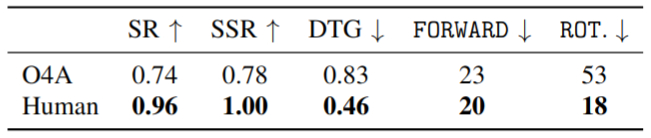O4A solves most episodes and achieves an average DTG of under 1m, even if most goals were not visible from the starting location and located up to 9 meters away.

## Embeddings

The geodesic regressor's training objective has strong connections with the manifold learning literature, and we show how the first 2 principal components of its last layer results in interpretable visualizations by comparing them to the ground truth maps. Points correspond to the location of RGB observations and are colored by the sum of their $x$ and $y$ coordinates. The unsupervised latent geometry is often consistent with the environment geometry, and some topological features (e.g., the obstacle "holes") are evident in the latent space, even if the training of O4A never used pose information.

Aloha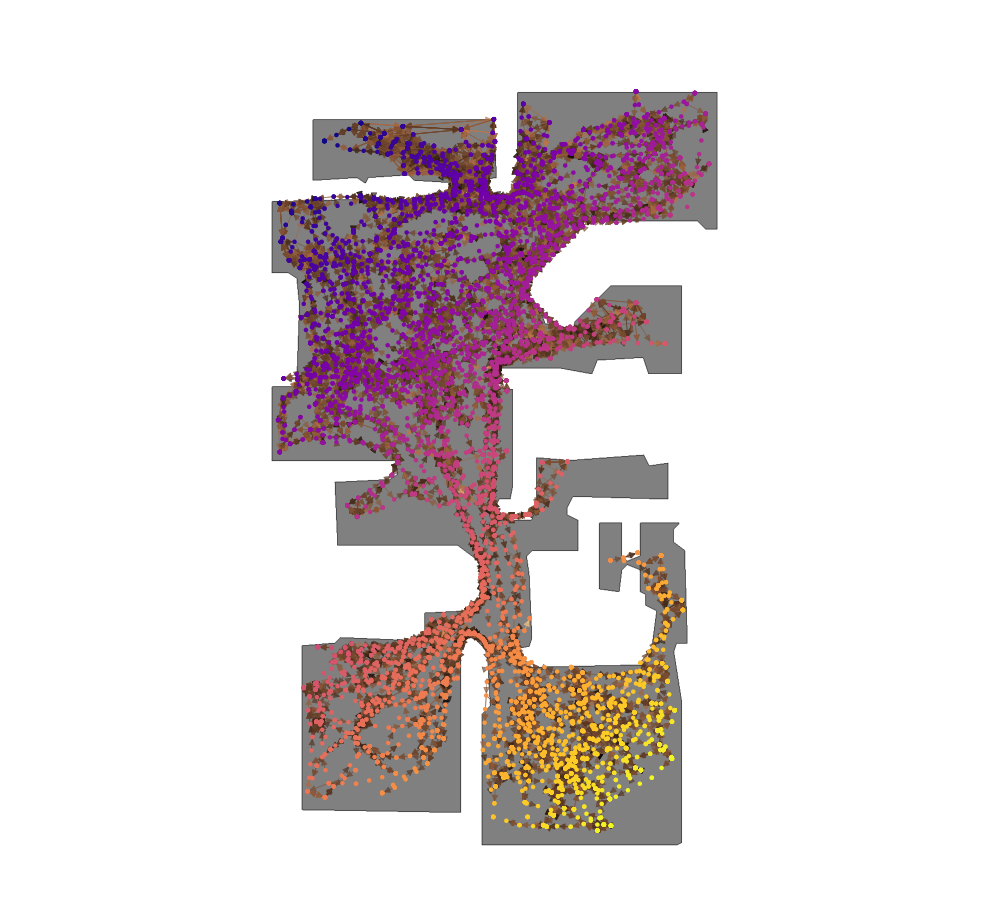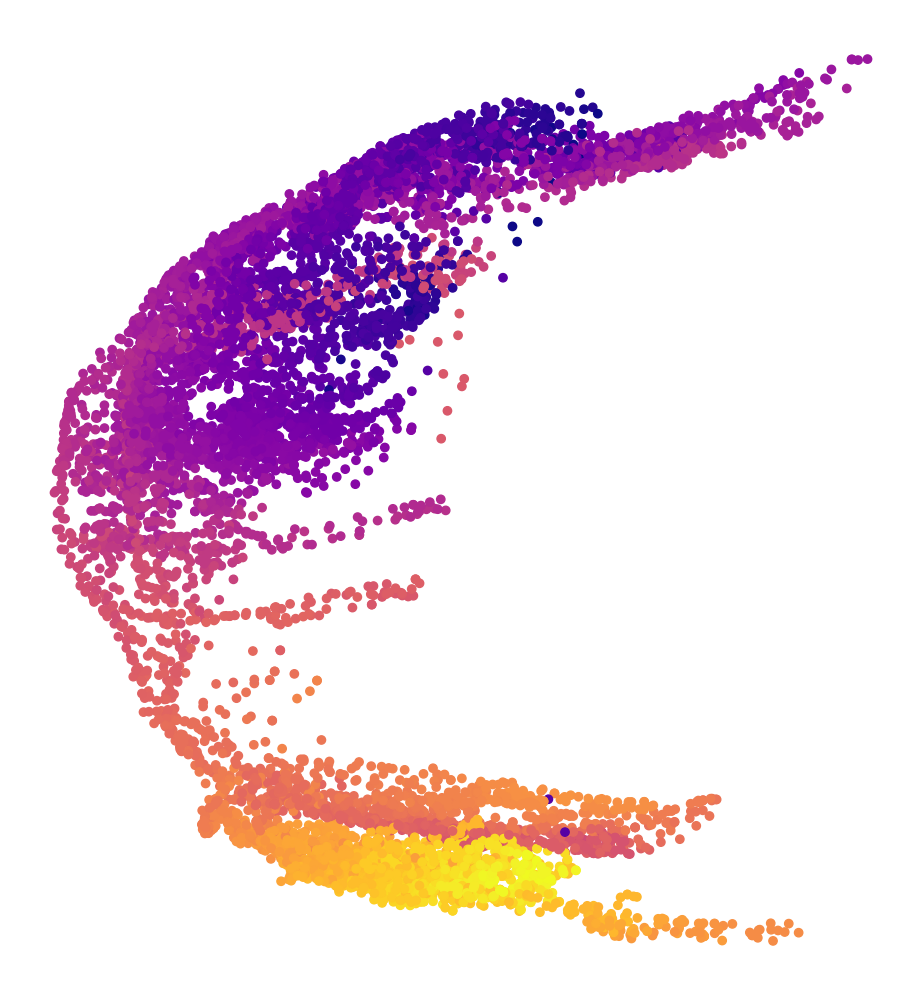Annawan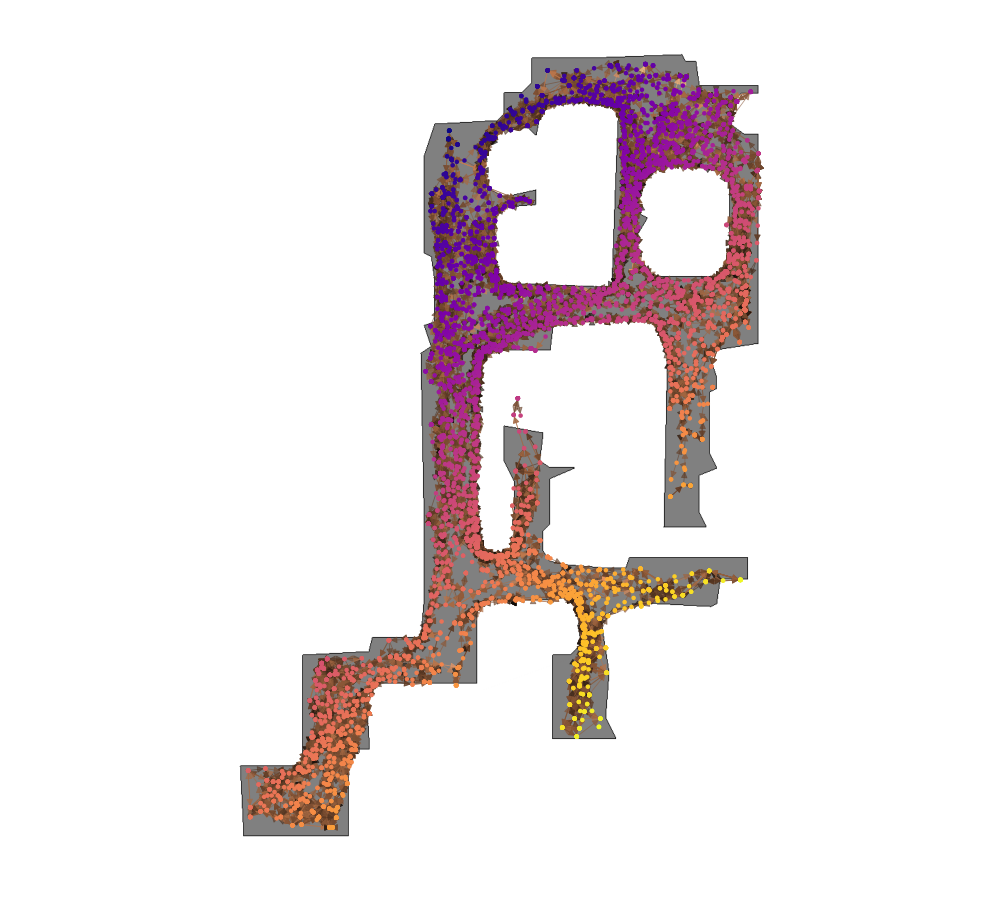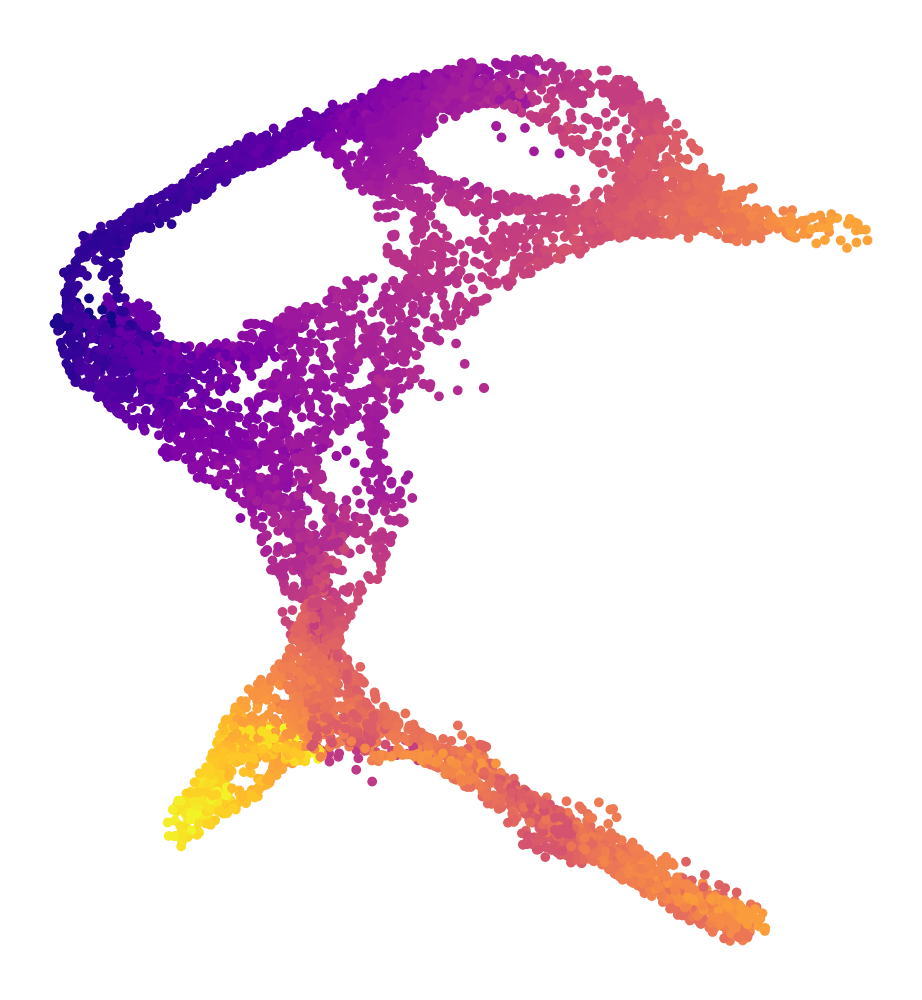Cantwell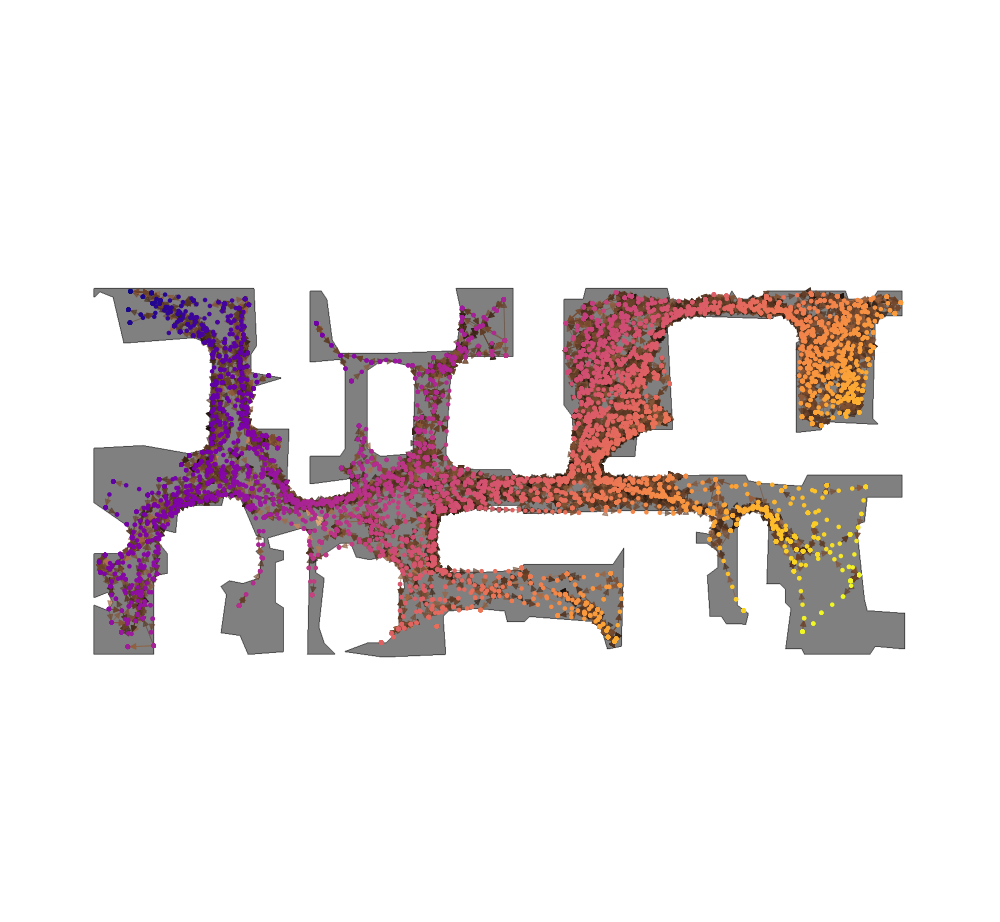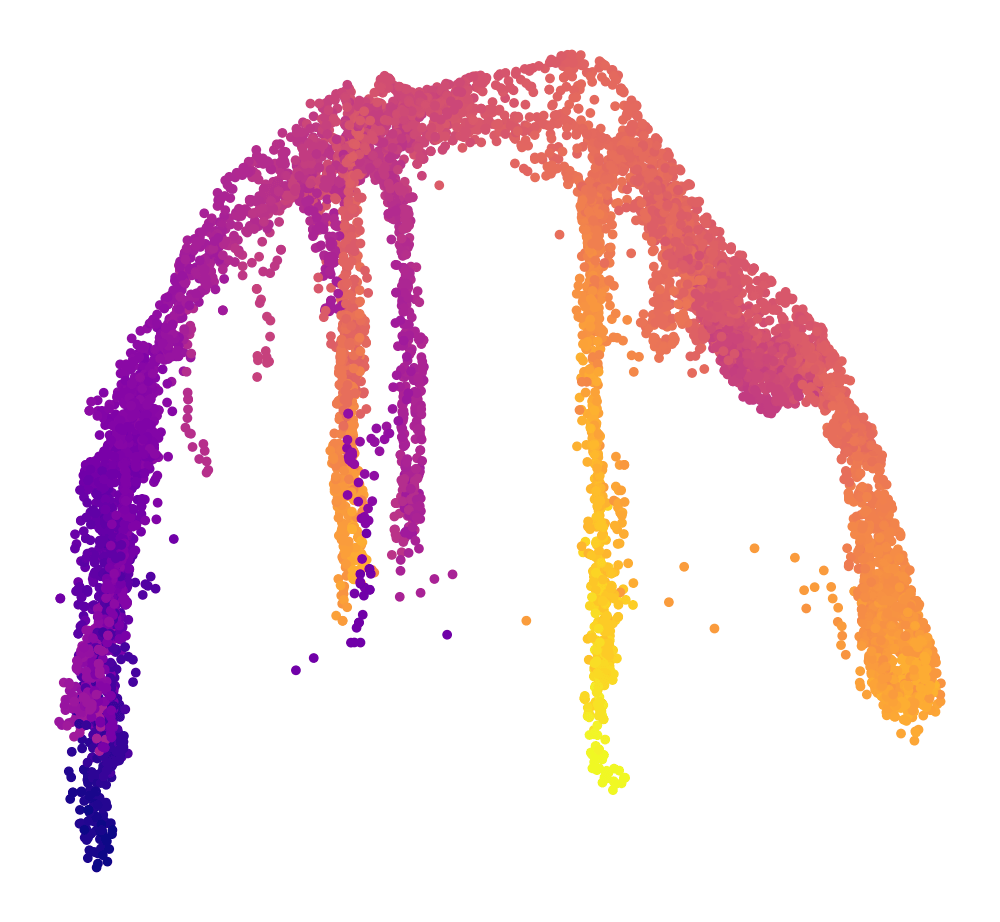Dunmor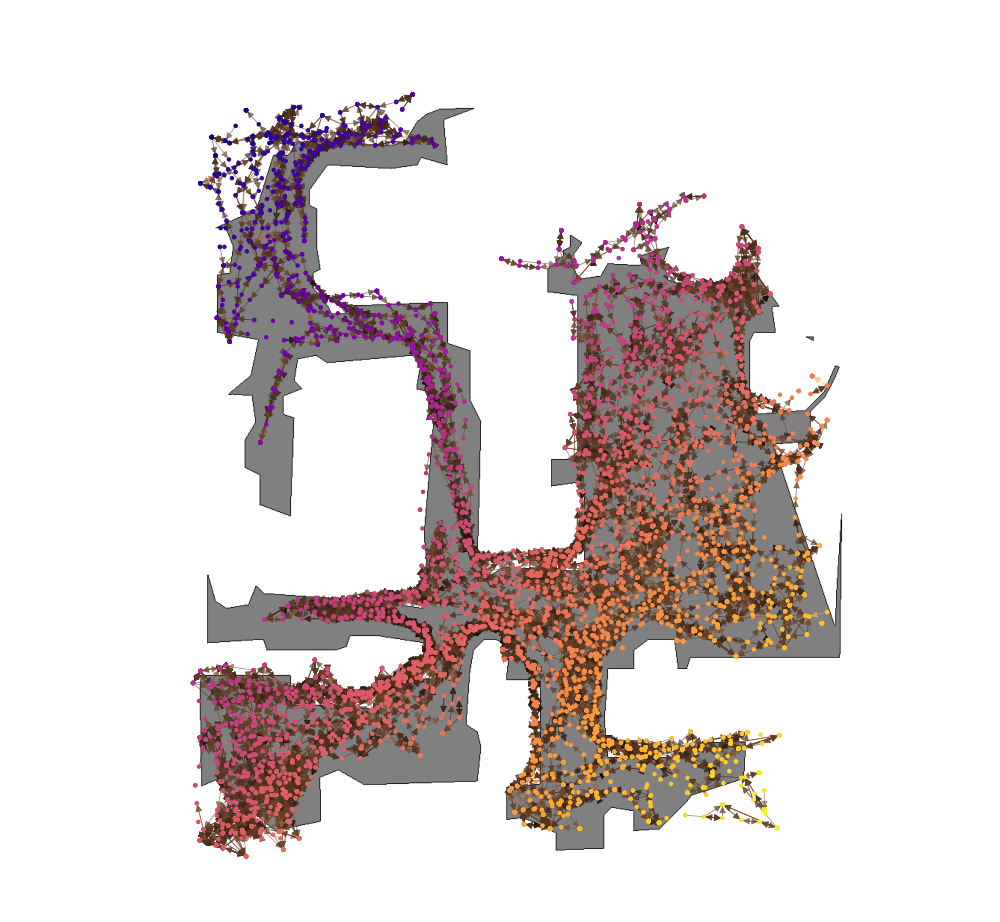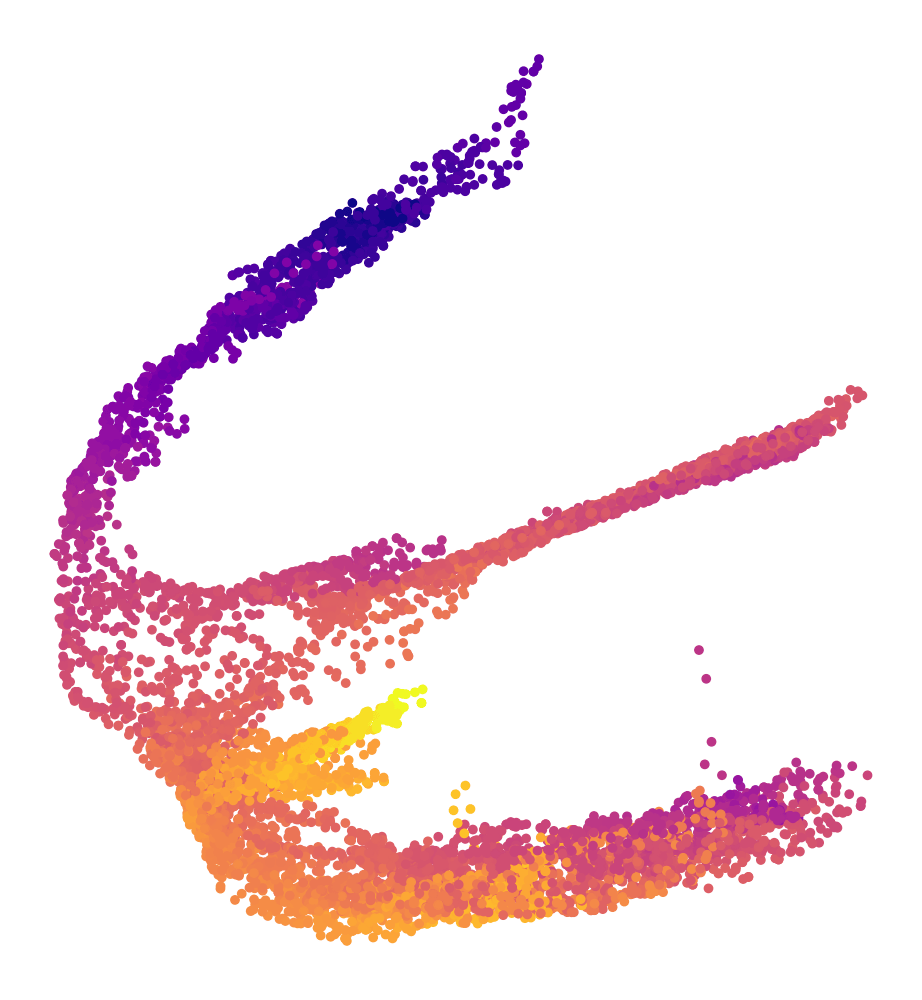Eastville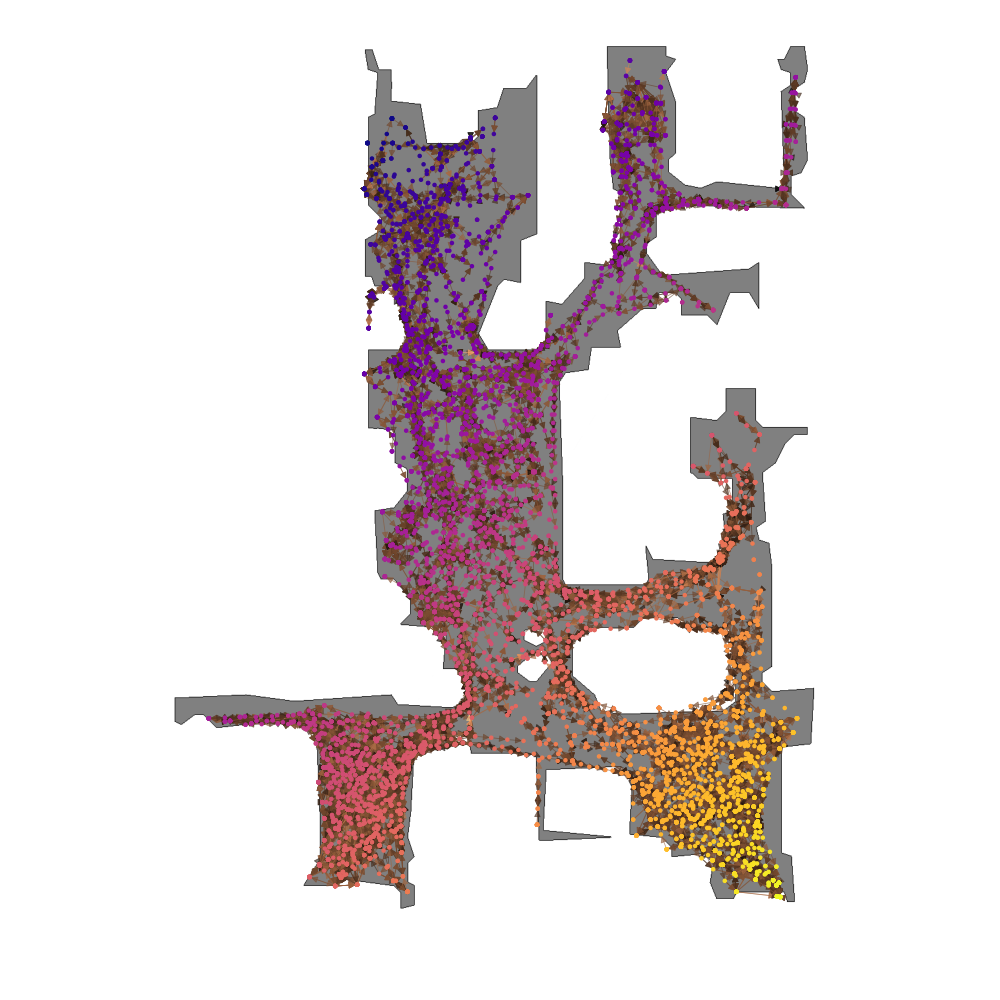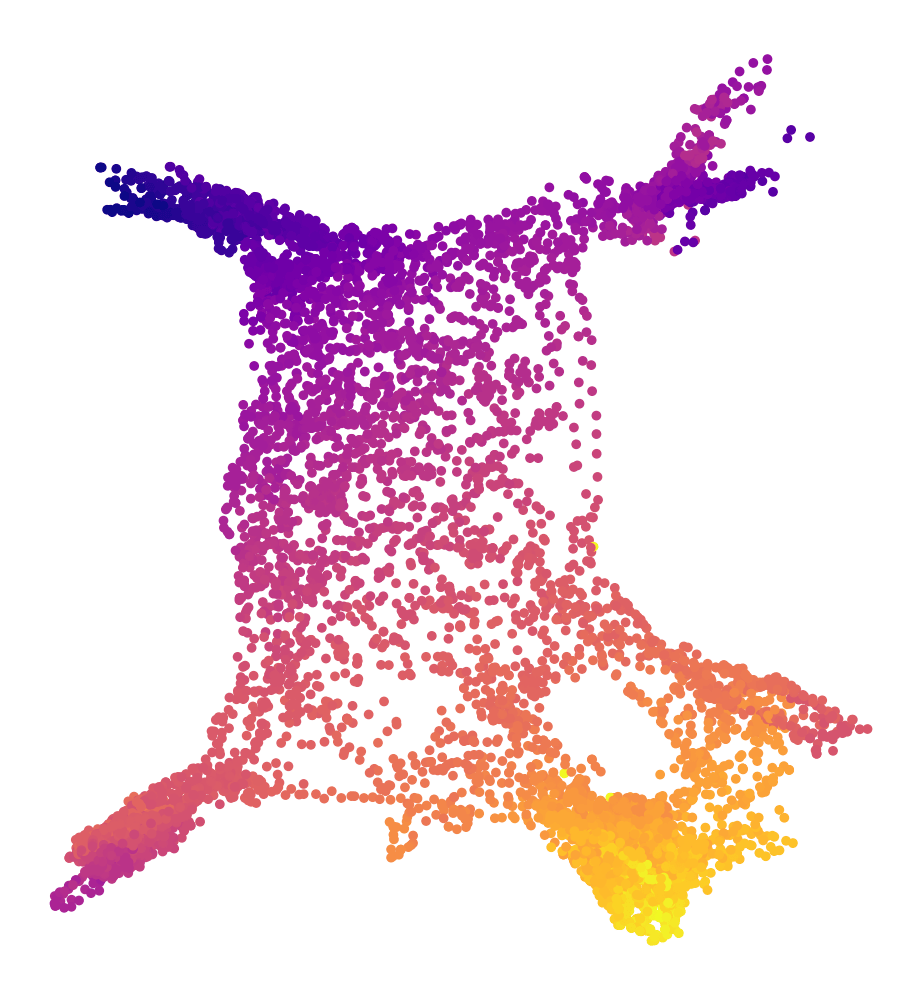Hambleton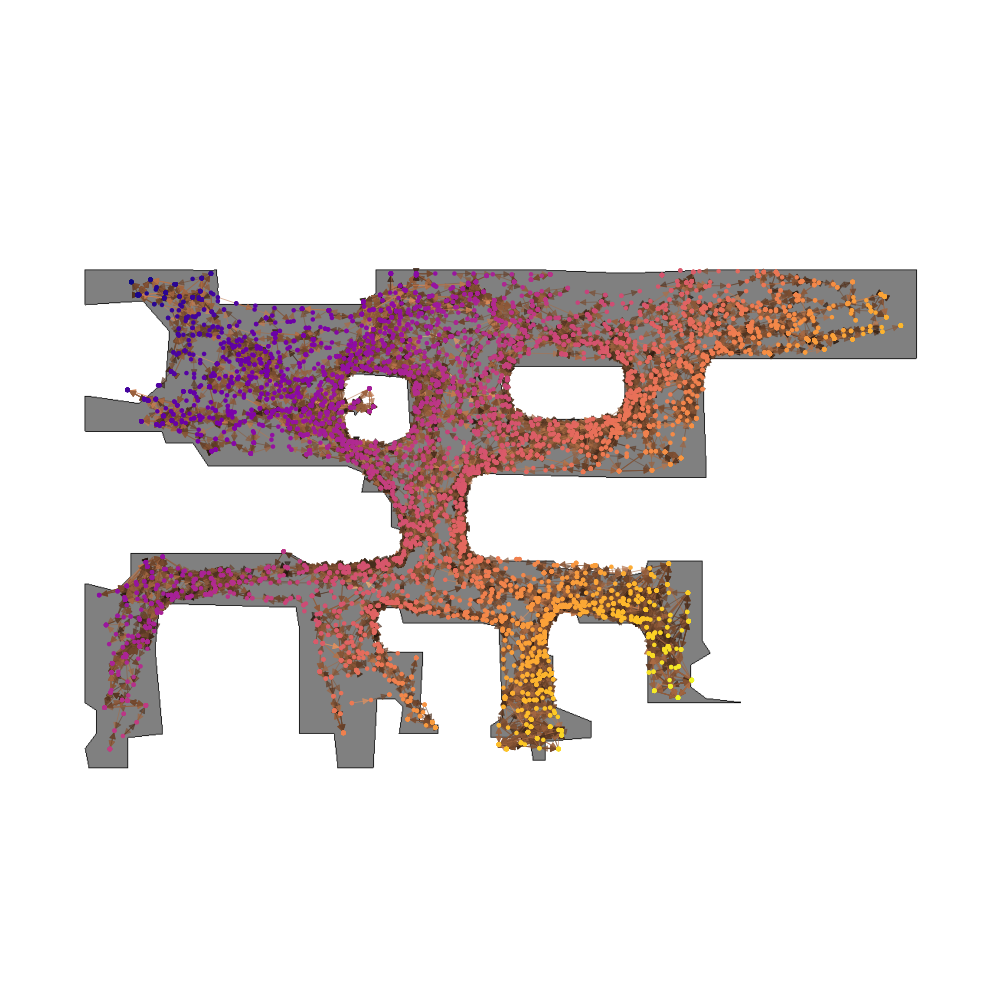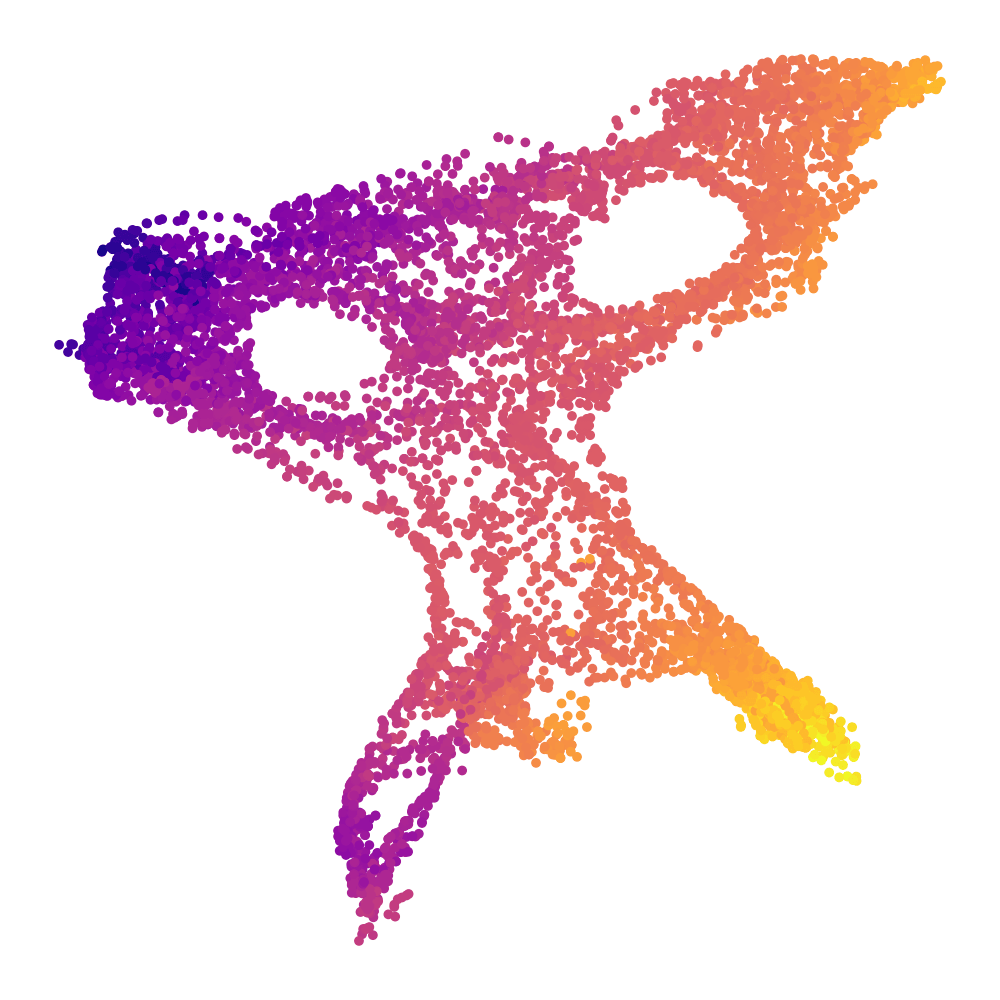Nicut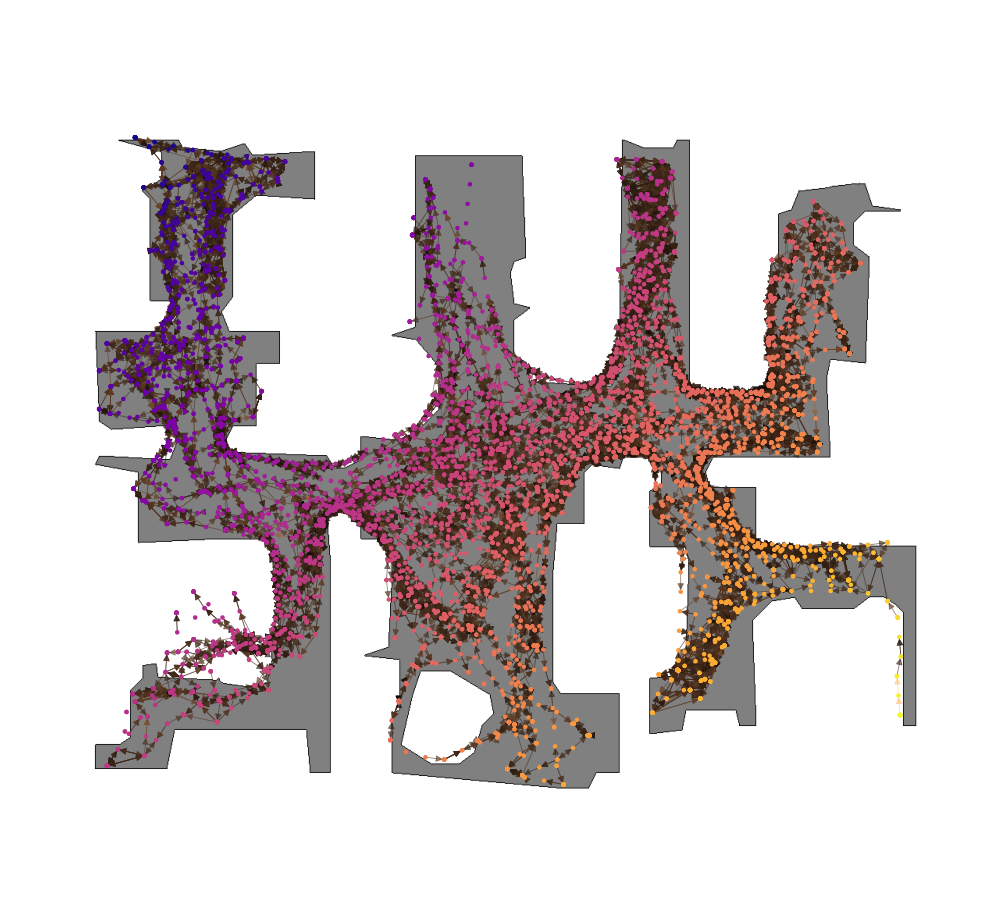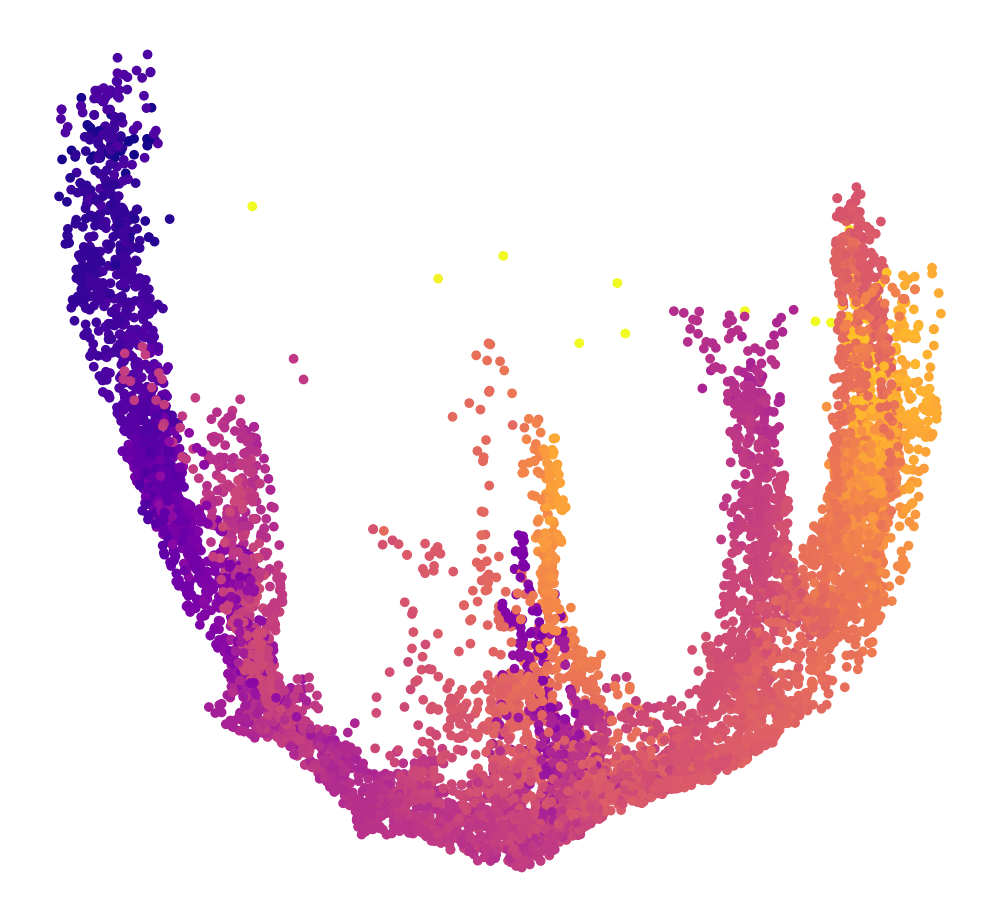Sodaville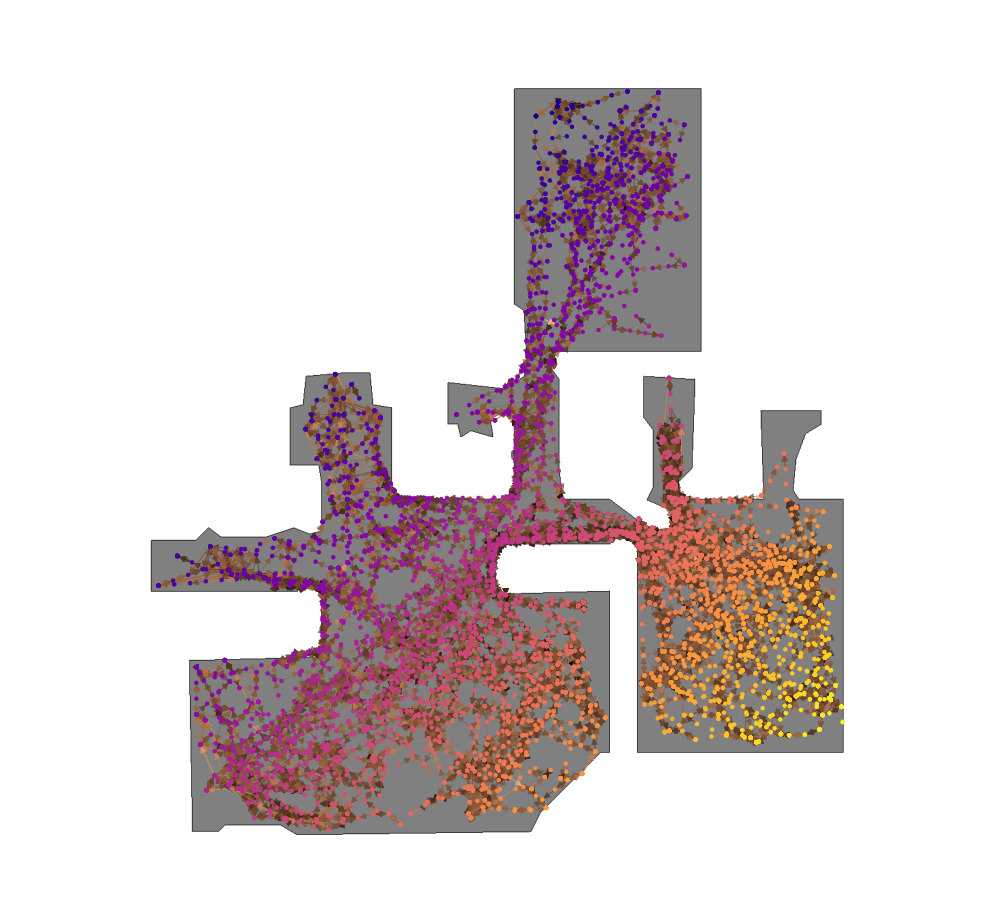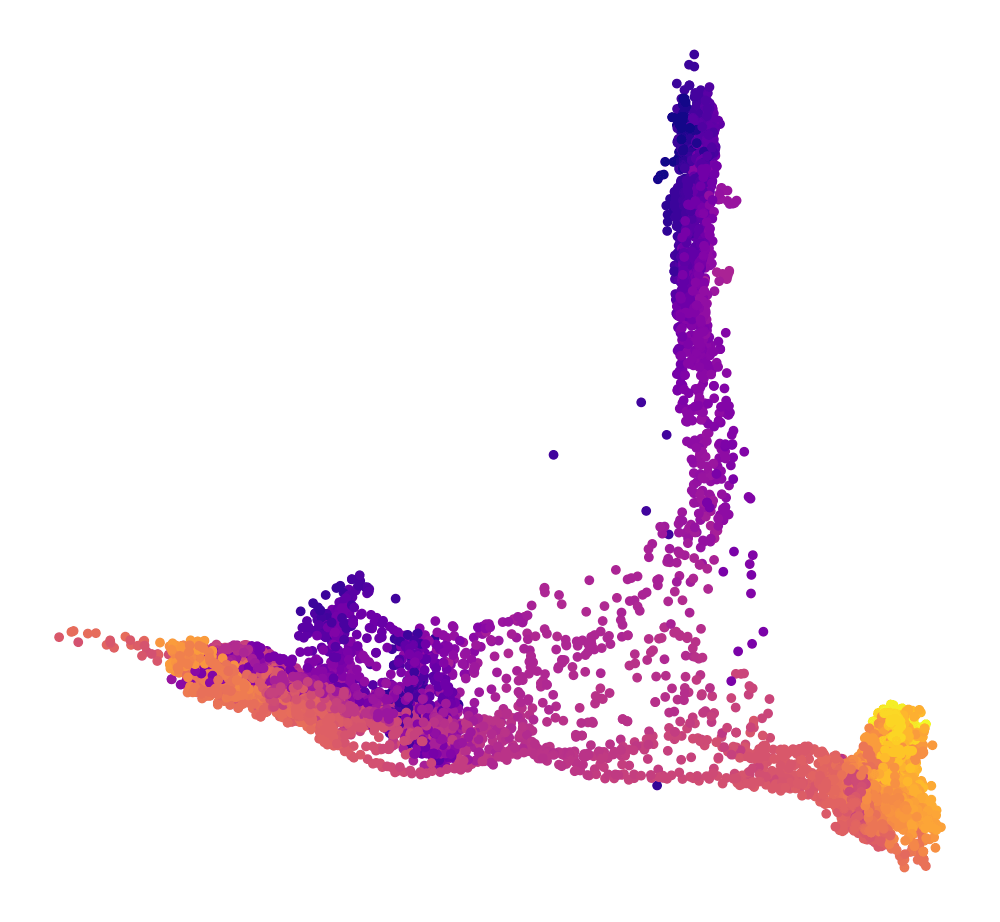## Detailed architectures and baselines

We finally show detailed architectures and hyperparameters for O4A and the baselines we used.

### O4A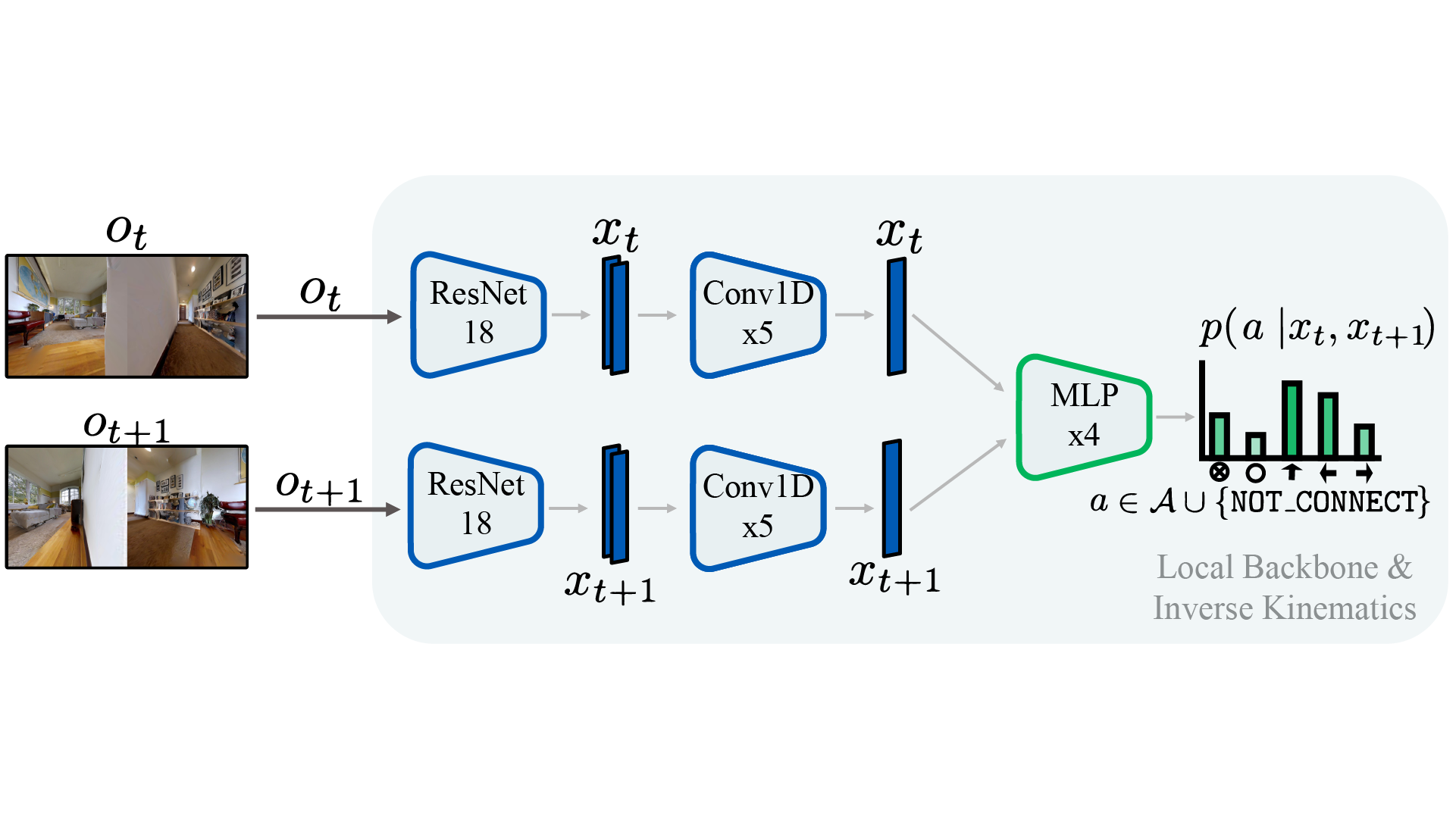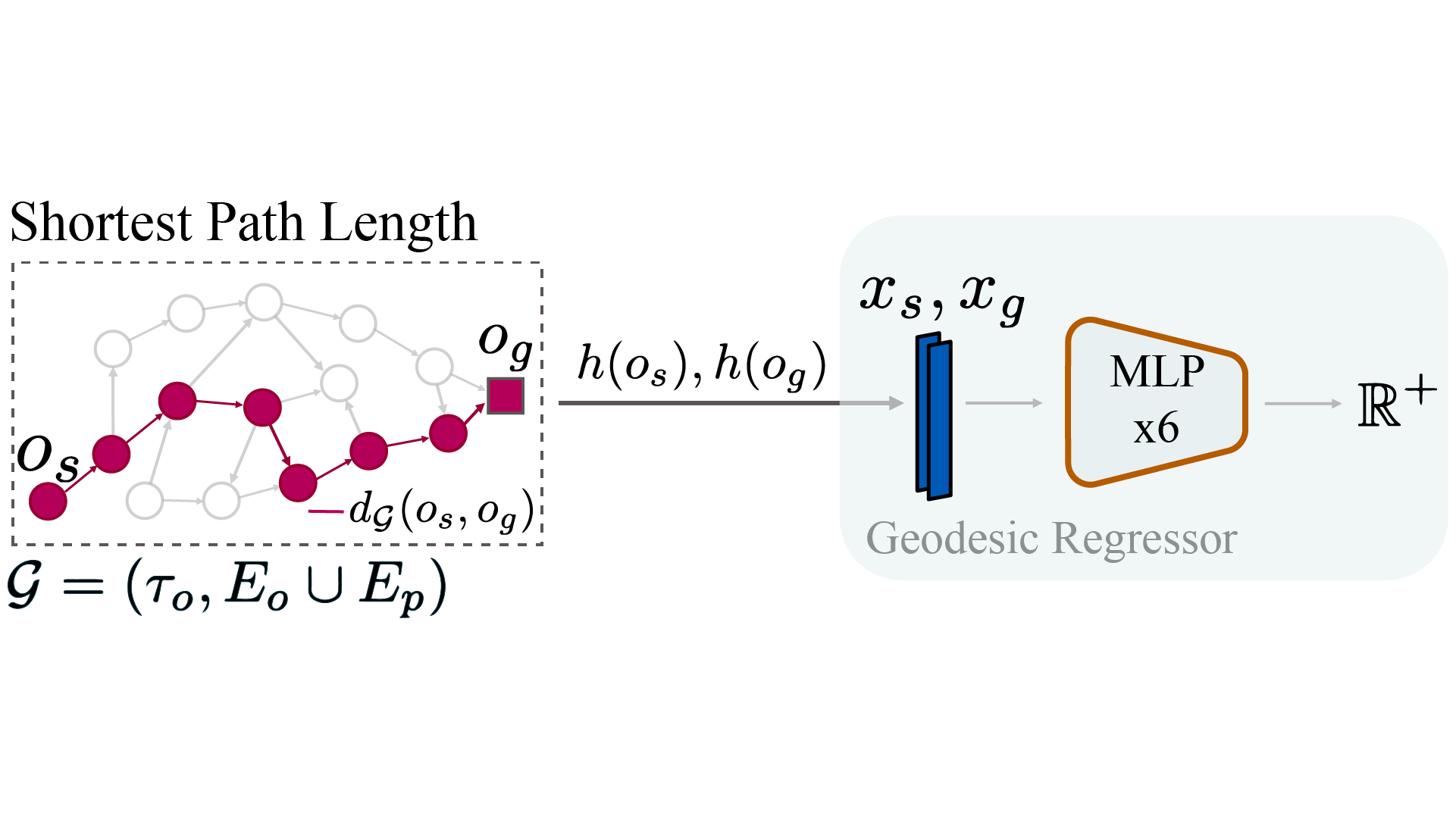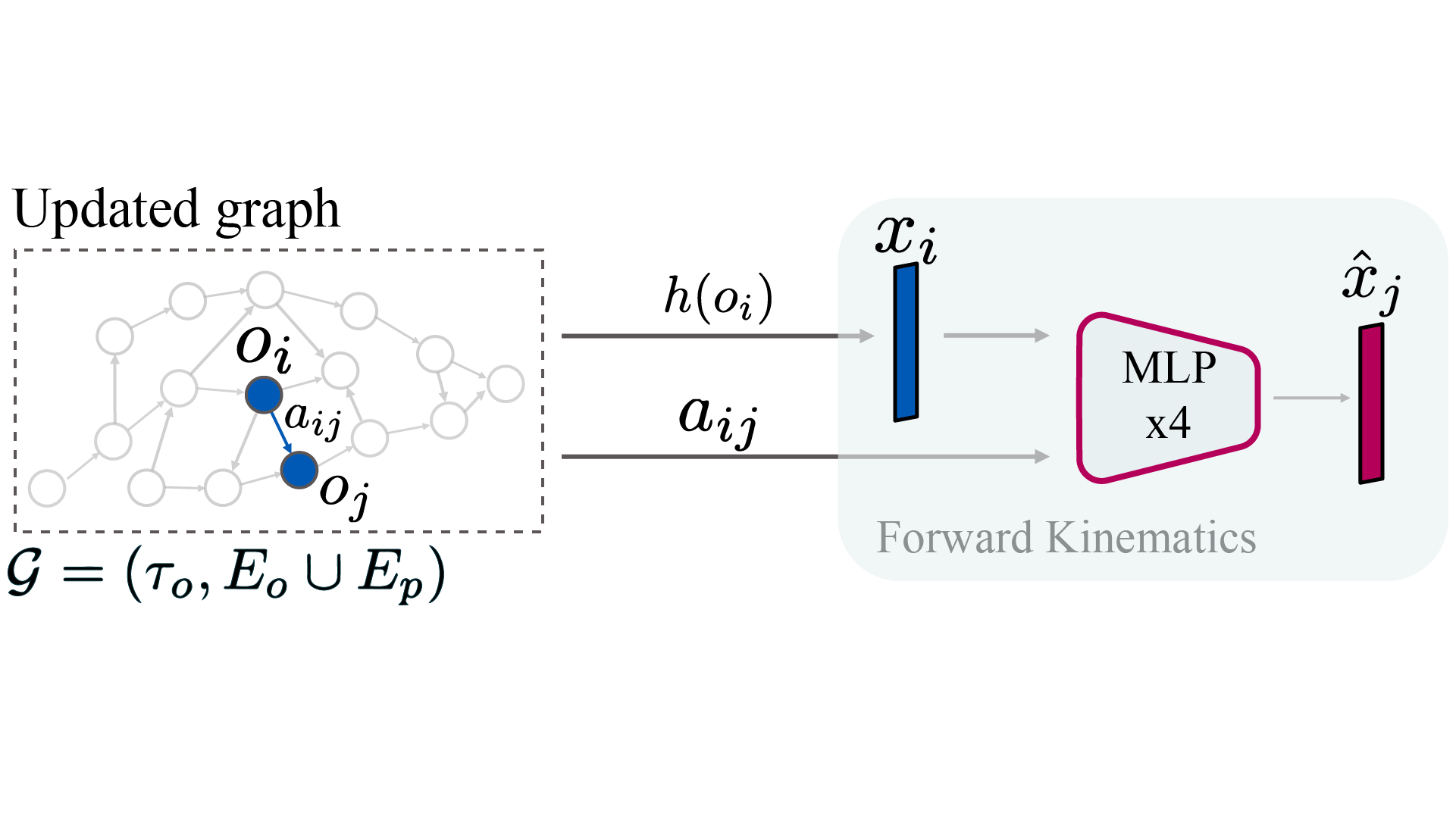### SPTM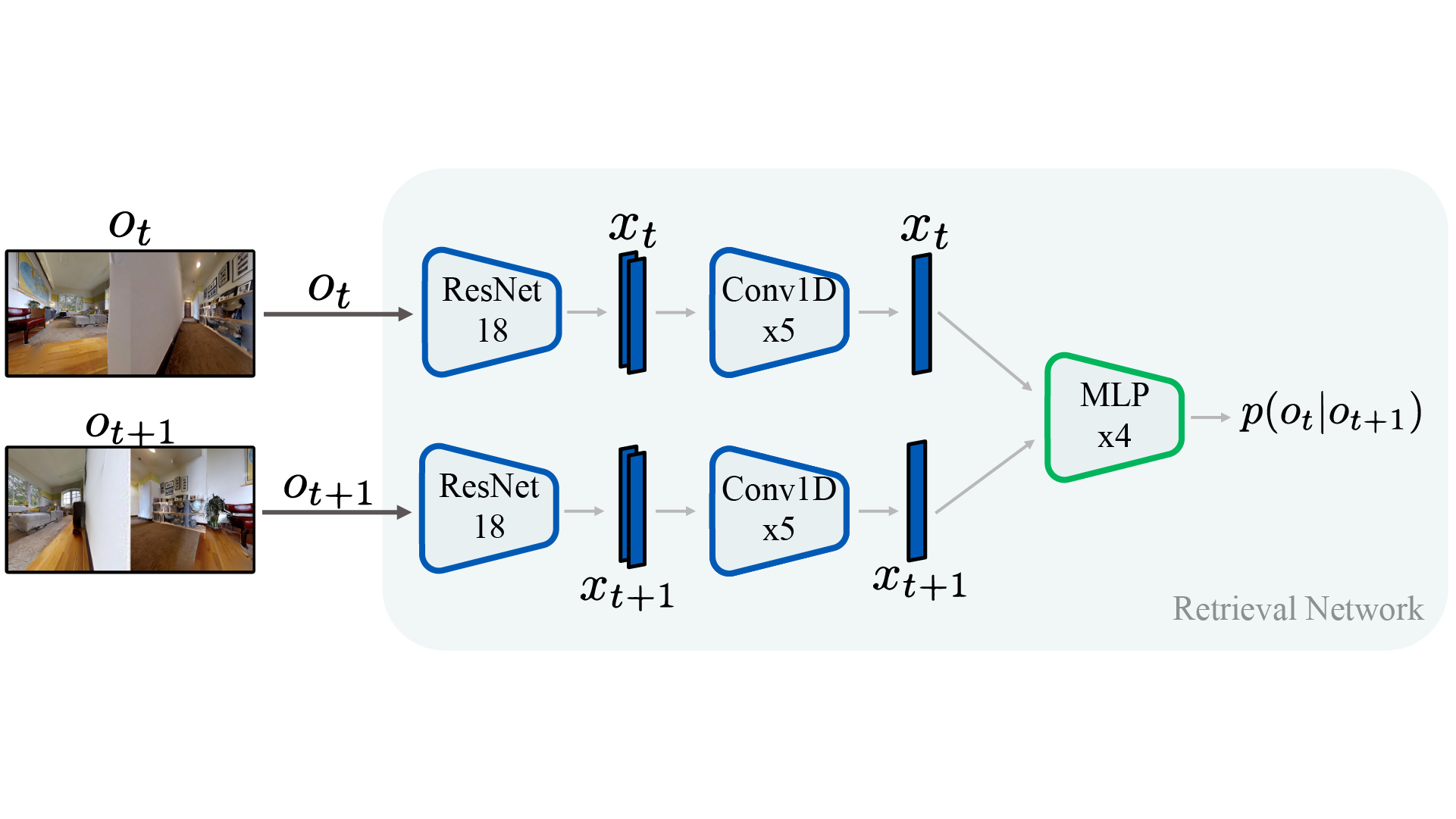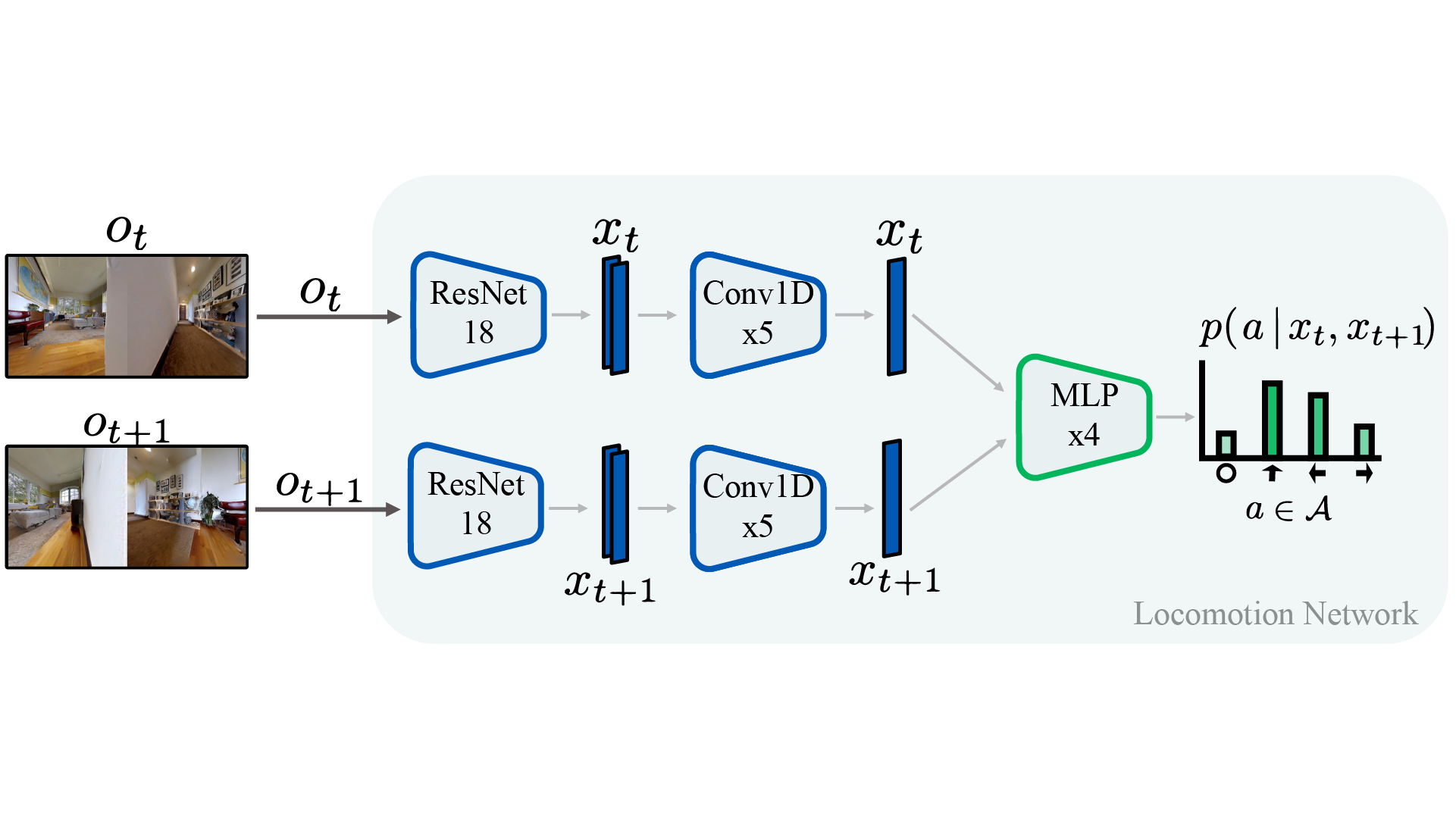### ViNG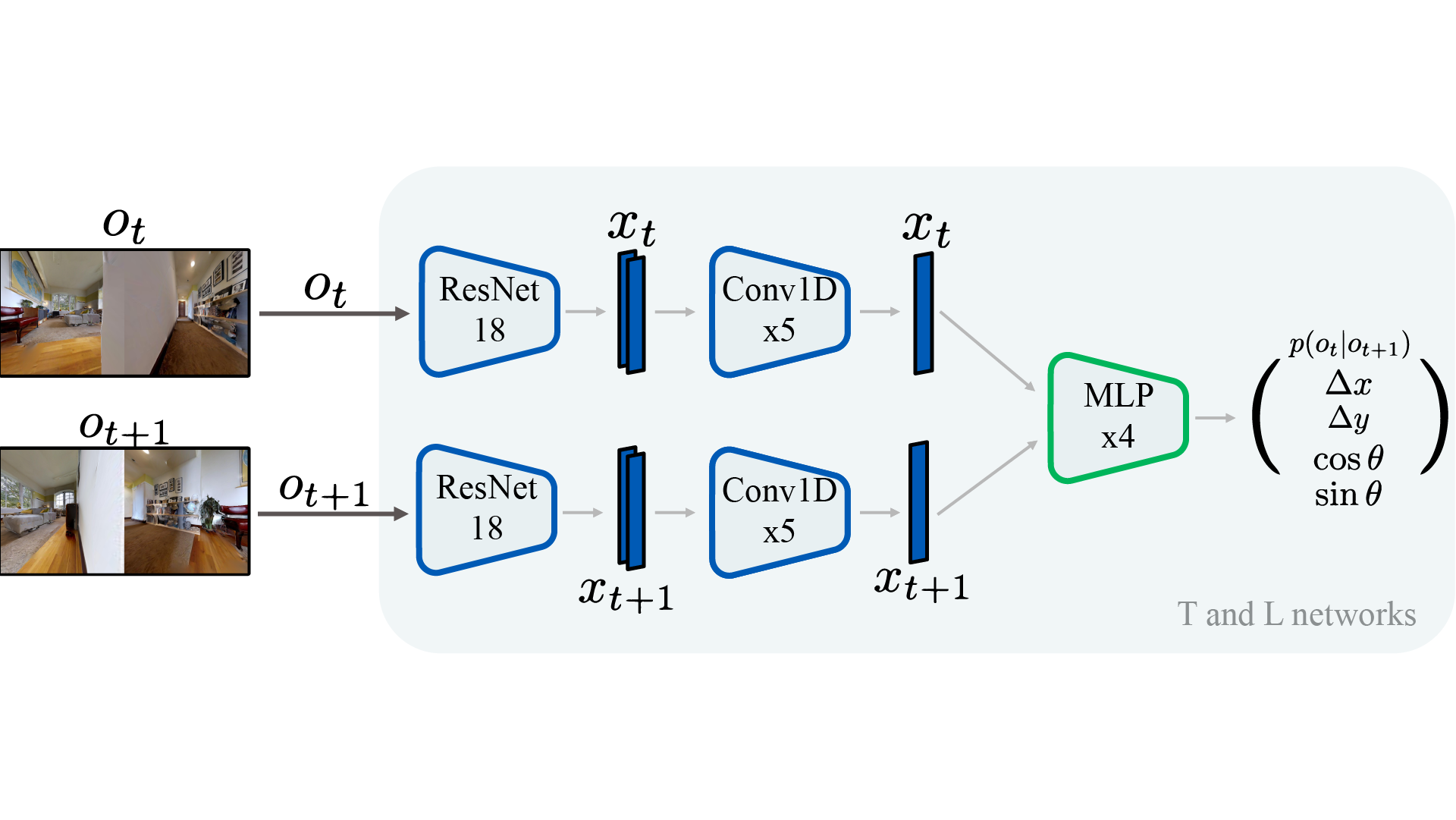### Hyperparameters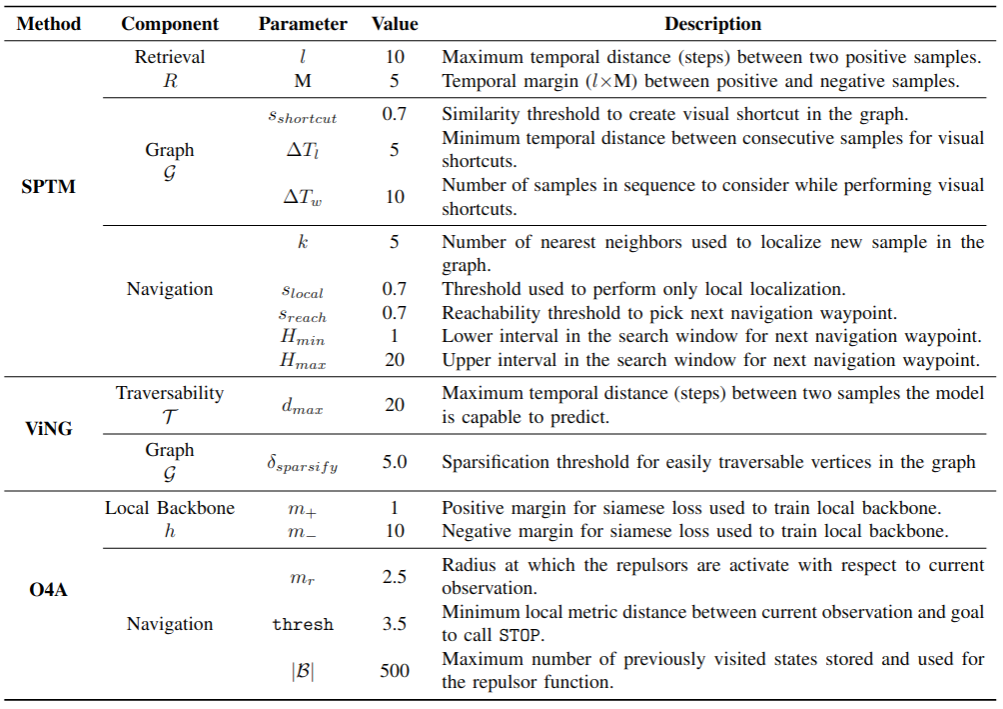## Extra plots for the Simulation Experiments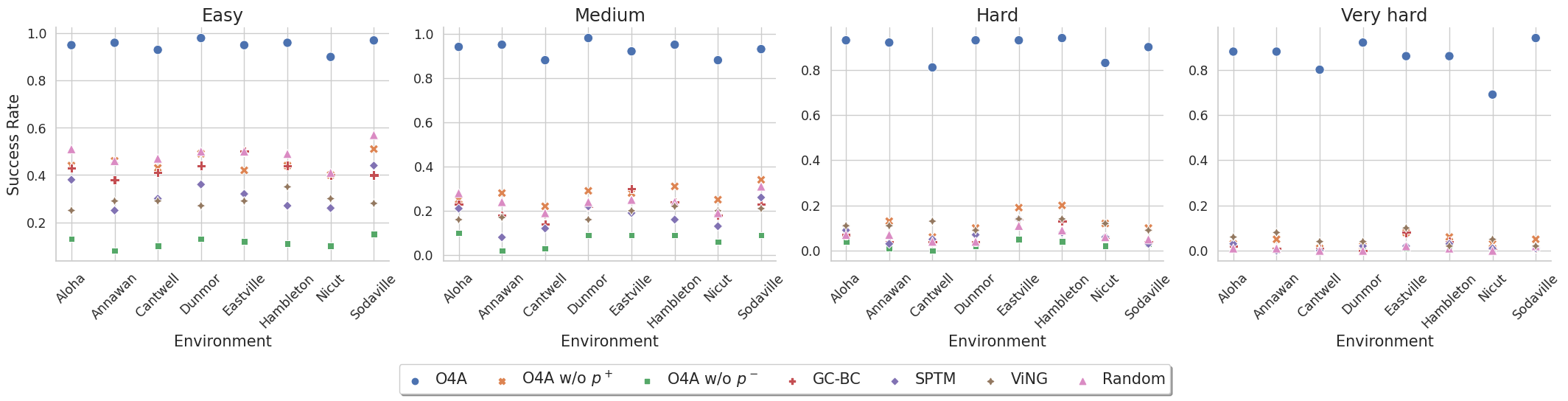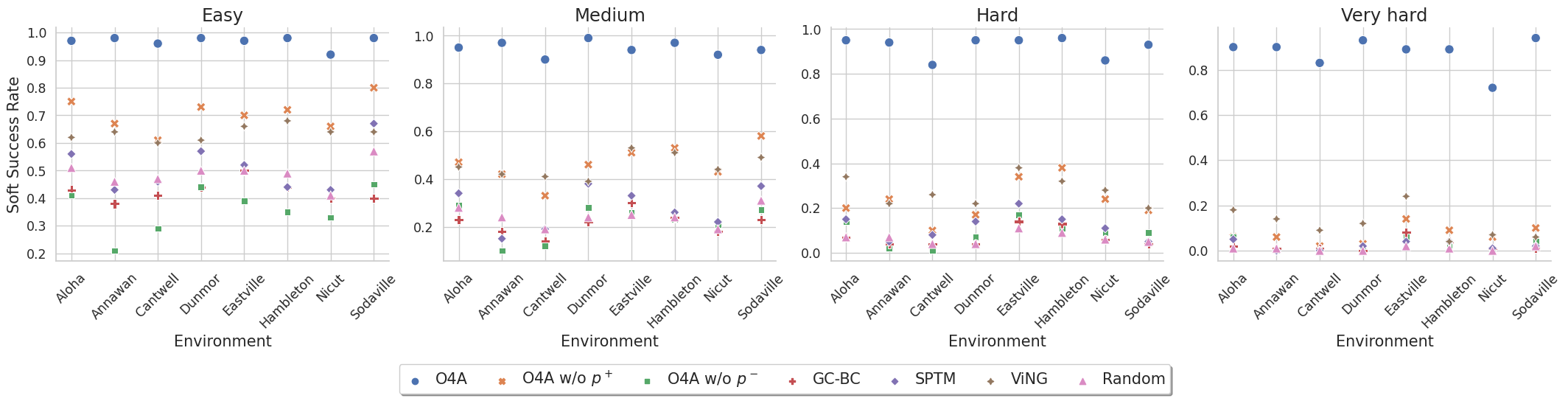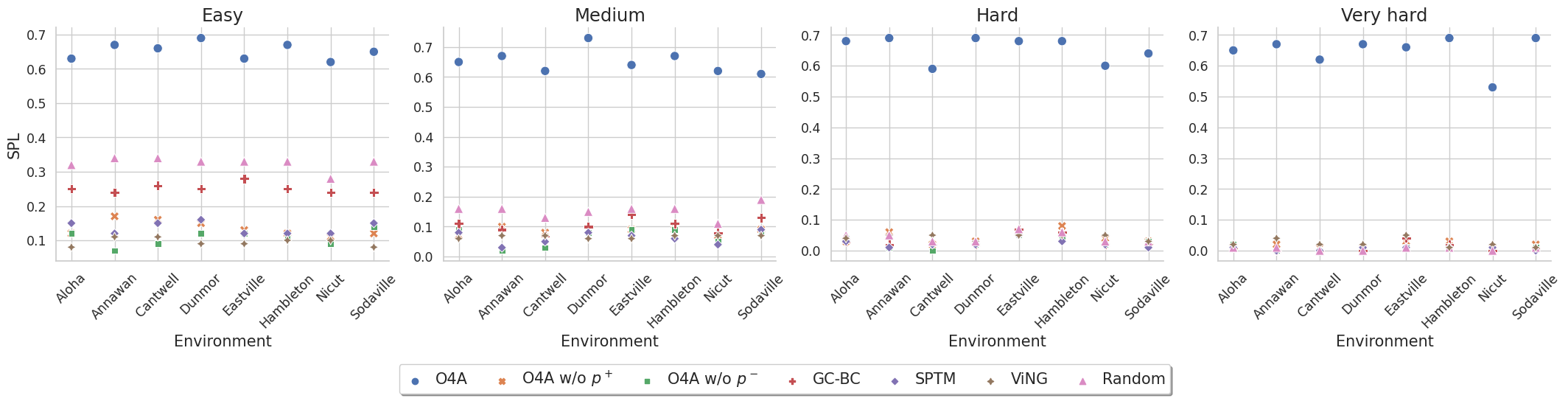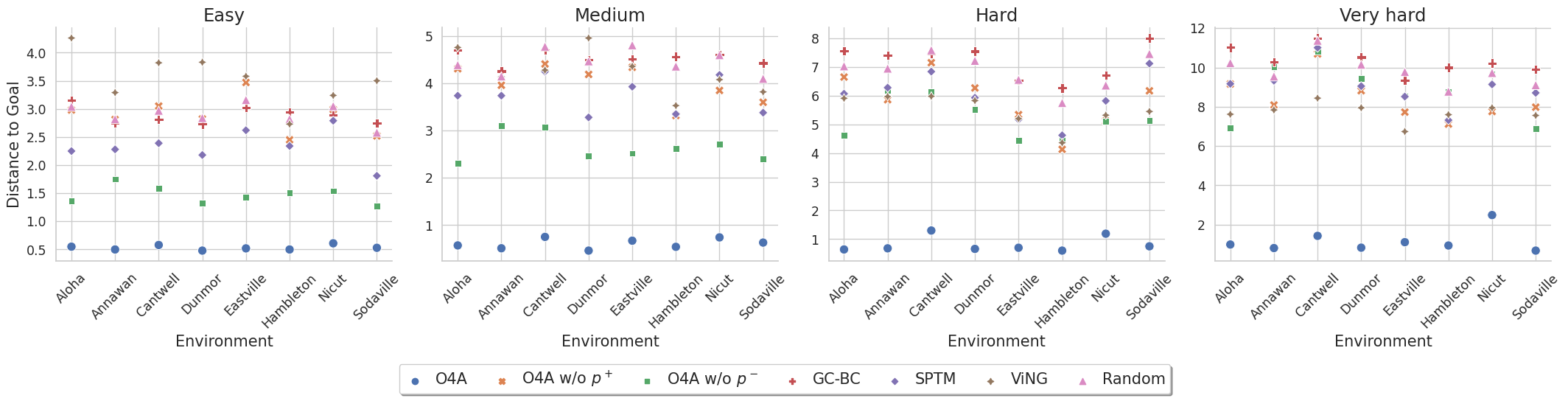## Augmentations used during Taining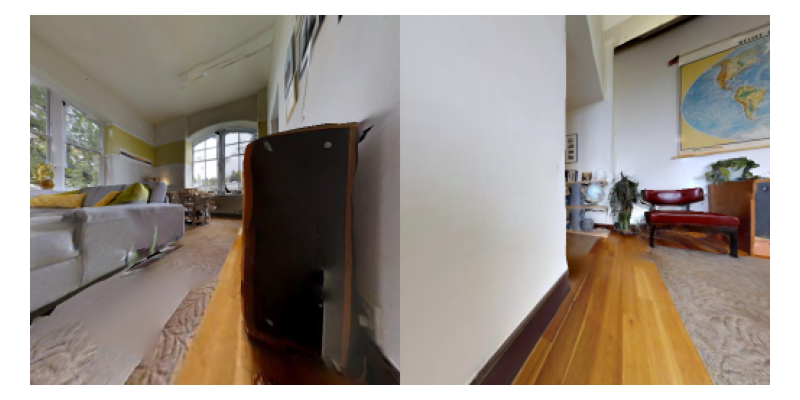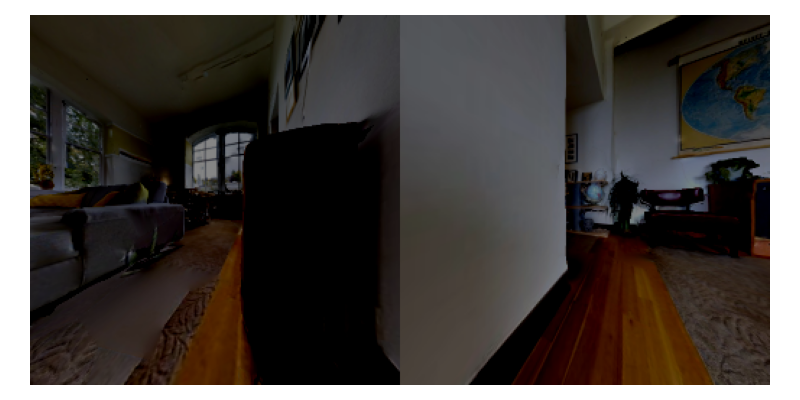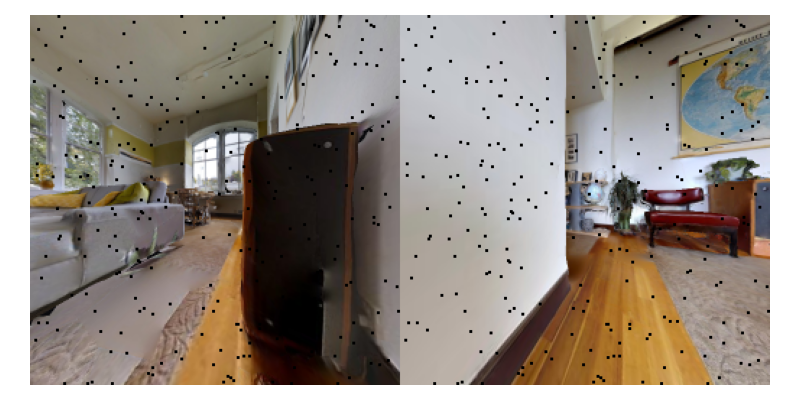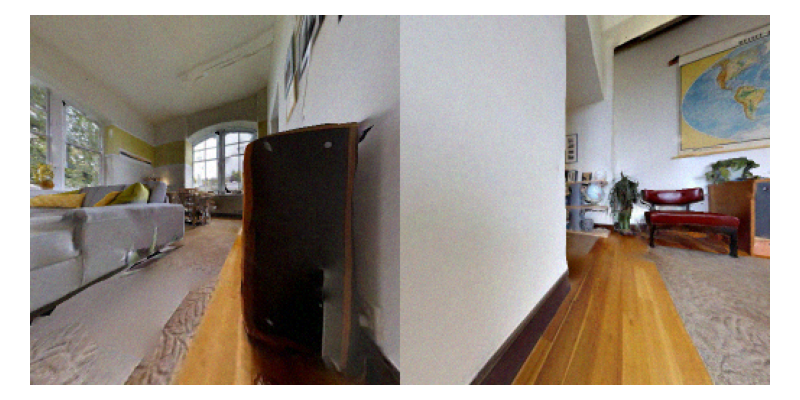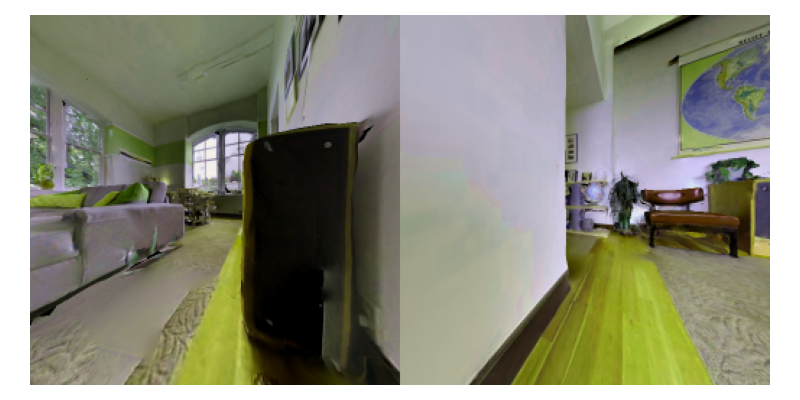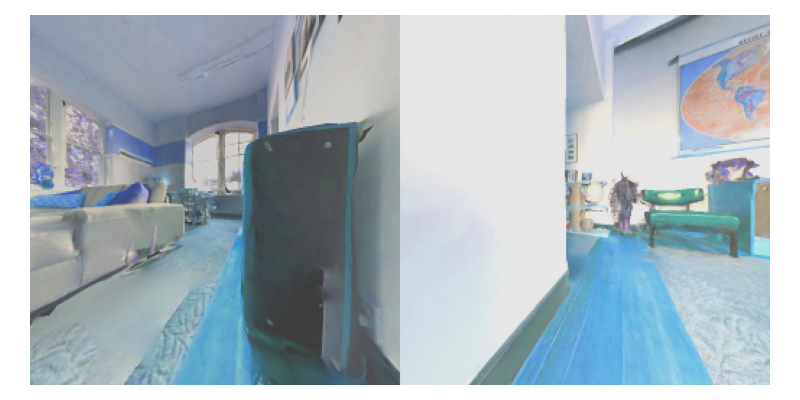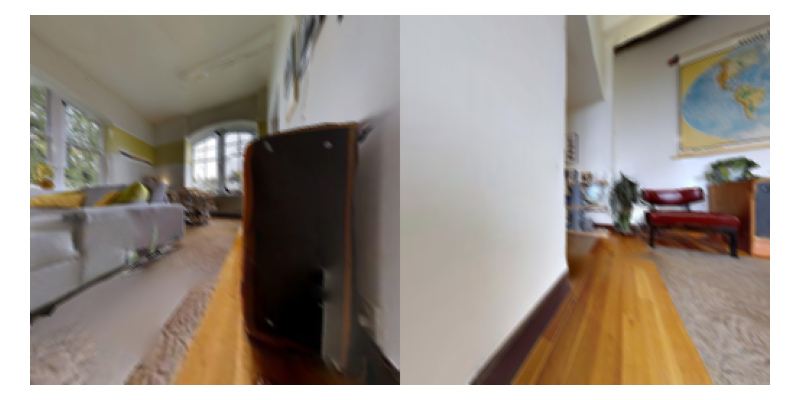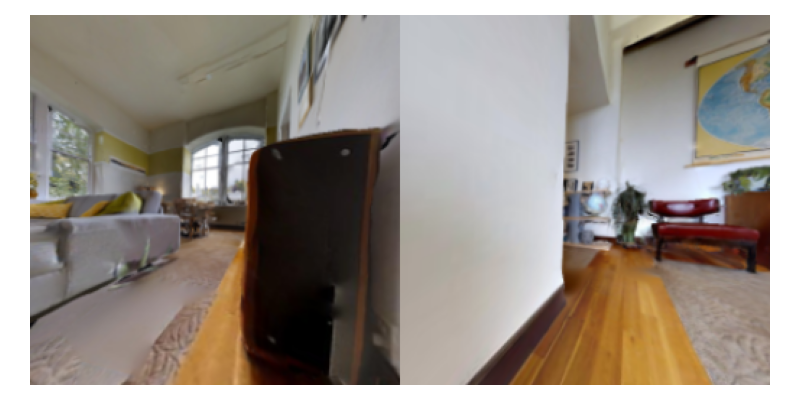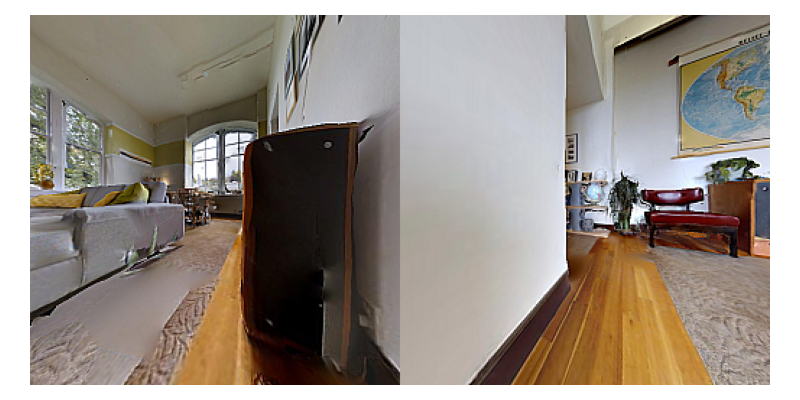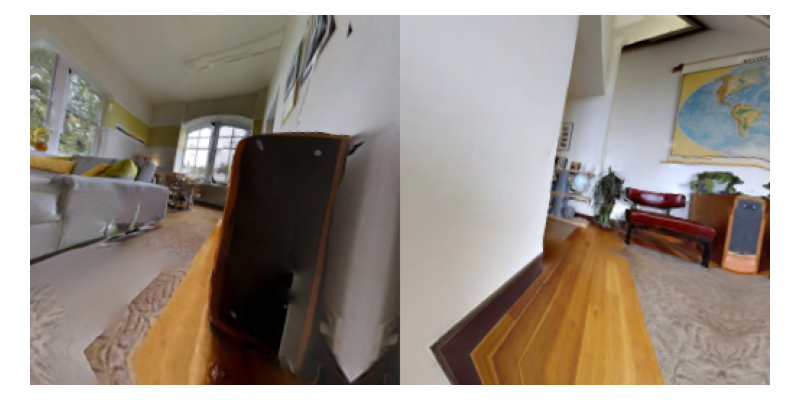## Citation

@article{morin2023one,
title        = {One-4-All: Neural Potential Fields for Embodied Navigation},
author       = {Morin, Sacha and Saavedra-Ruiz, Miguel and Paull, Liam},
year         = 2023,
journal      = {arXiv preprint arXiv:2303.04011}
}